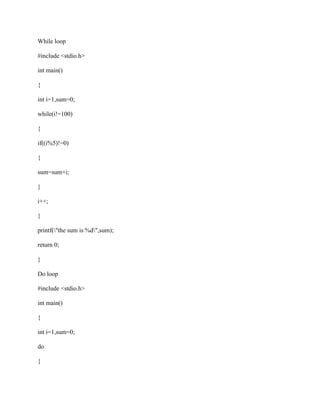Successfully reported this slideshow.

# Write a for loop to sum the number not divisible by 5 in the range fro.docx×

# Write a for loop to sum the number not divisible by 5 in the range fro.docx

Write a for loop to sum the number not divisible by 5 in the range from 1 to 100. Print the sum after the loop is complete. Write a do loop to sum the numbers not divisible by 5 in the range from 1 to 100. Print the sum after the loop is complete. Write a while loop to sum the numbers not divisible by 5 in the range from 1 to 100. Print the sum after the loop is complete.
Solution
For loop
#include <stdio.h>
int main()
{
int i,sum=0;
for(i=1;i<=100;i++)
{
if((i%5)!=0)
{
sum=sum+i;
}
}
printf(\"the sum is %d\",sum);
return 0;
}
While loop
#include <stdio.h>
int main()
{
int i=1,sum=0;
while(i!=100)
{
if((i%5)!=0)
{
sum=sum+i;
}
i++;
}
printf(\"the sum is %d\",sum);
return 0;
}
Do loop
#include <stdio.h>
int main()
{
int i=1,sum=0;
do
{
if((i%5)!=0)
{
sum=sum+i;
}
i++;
}while(i!=100);
printf(\"the sum is %d\",sum);
return 0;
}
.

Write a for loop to sum the number not divisible by 5 in the range from 1 to 100. Print the sum after the loop is complete. Write a do loop to sum the numbers not divisible by 5 in the range from 1 to 100. Print the sum after the loop is complete. Write a while loop to sum the numbers not divisible by 5 in the range from 1 to 100. Print the sum after the loop is complete.
Solution
For loop
#include <stdio.h>
int main()
{
int i,sum=0;
for(i=1;i<=100;i++)
{
if((i%5)!=0)
{
sum=sum+i;
}
}
printf(\"the sum is %d\",sum);
return 0;
}
While loop
#include <stdio.h>
int main()
{
int i=1,sum=0;
while(i!=100)
{
if((i%5)!=0)
{
sum=sum+i;
}
i++;
}
printf(\"the sum is %d\",sum);
return 0;
}
Do loop
#include <stdio.h>
int main()
{
int i=1,sum=0;
do
{
if((i%5)!=0)
{
sum=sum+i;
}
i++;
}while(i!=100);
printf(\"the sum is %d\",sum);
return 0;
}
.